# Ytl's Java Blog

摘要: 欧几里德算法  阅读全文

posted @ 2013-03-21 09:39 ytl 阅读(281) | 评论 (0)编辑 收藏

# Quicksort

Quicksort is a fast sorting algorithm, which is used not only for educational purposes, but widely applied in practice. On the average, it has O(n log n) complexity, making quicksort suitable for sorting big data volumes. The idea of the algorithm is quite simple and once you realize it, you can write quicksort as fast as bubble sort.

## Algorithm

The divide-and-conquer strategy is used in quicksort. Below the recursion step is described:
1. Choose a pivot value. We take the value of the middle element as pivot value, but it can be any value, which is in range of sorted values, even if it doesn't present in the array.
2. Partition. Rearrange elements in such a way, that all elements which are lesser than the pivot go to the left part of the array and all elements greater than the pivot, go to the right part of the array. Values equal to the pivot can stay in any part of the array. Notice, that array may be divided in non-equal parts.
3. Sort both parts. Apply quicksort algorithm recursively to the left and the right parts.

### Partition algorithm in detail

There are two indices i and j and at the very beginning of the partition algorithm i points to the first element in the array andj points to the last one. Then algorithm moves i forward, until an element with value greater or equal to the pivot is found. Index j is moved backward, until an element with value lesser or equal to the pivot is found. If i ≤ j then they are swapped and i steps to the next position (i + 1), j steps to the previous one (j - 1). Algorithm stops, when i becomes greater than j.

After partition, all values before i-th element are less or equal than the pivot and all values after j-th element are greater or equal to the pivot.

Example. Sort {1, 12, 5, 26, 7, 14, 3, 7, 2} using quicksort.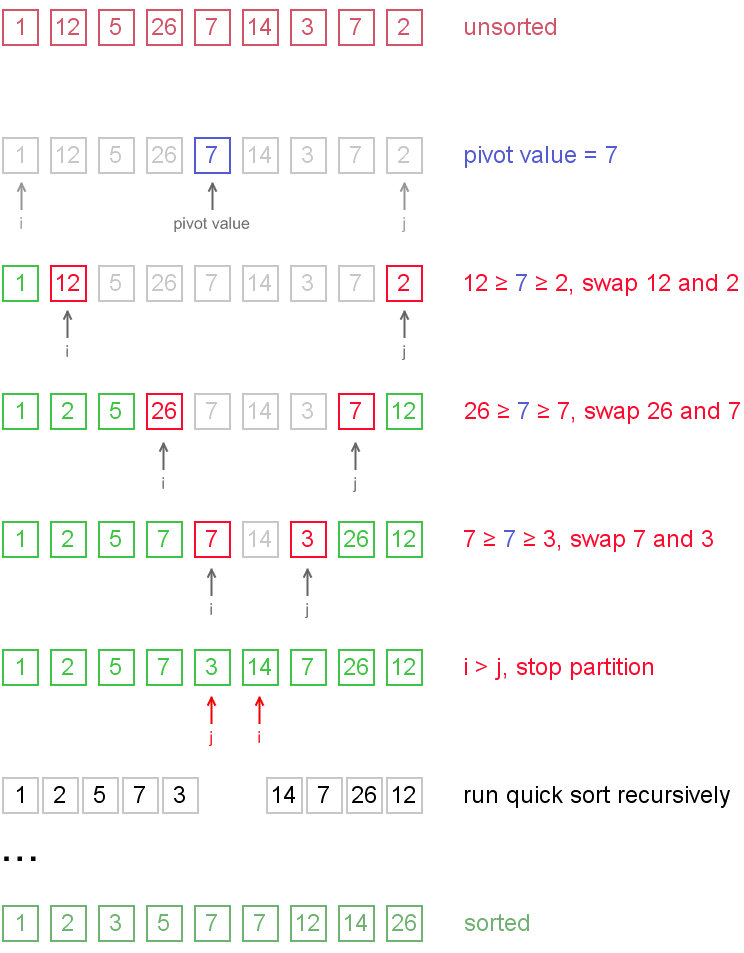Notice, that we show here only the first recursion step, in order not to make example too long. But, in fact, {1, 2, 5, 7, 3} and {14, 7, 26, 12} are sorted then recursively.

## Why does it work?

On the partition step algorithm divides the array into two parts and every element a from the left part is less or equal than every element b from the right part. Also a and b satisfy a ≤ pivot ≤ b inequality. After completion of the recursion calls both of the parts become sorted and, taking into account arguments stated above, the whole array is sorted.

## Complexity analysis

On the average quicksort has O(n log n) complexity, but strong proof of this fact is not trivial and not presented here. Still, you can find the proof in . In worst case, quicksort runs O(n2) time, but on the most "practical" data it works just fine and outperforms other O(n log n) sorting algorithms.

## Code snippets

### Java

int partition(int arr[], int left, int right)

{

int i = left;

int j = right;

int temp;

int  pivot = arr[(left+right)>>1];

while(i<=j){

while(arr[i]>=pivot){

i++;

}

while(arr[j]<=pivot){

j--;

}

if(i<=j){

temp = arr[i];

arr[i] = arr[j];

arr[j] = temp;

i++;

j--;

}

}

return i

}

void quickSort(int arr[], int left, int right) {

int index = partition(arr, left, right);

if(left<index-1){

quickSort(arr,left,index-1);

}

if(index<right){

quickSort(arr,index,right);

}

}

### python

def quickSort(L,left,right) {

i = left

j = right

if right-left <=1:

return L

pivot = L[(left + right) >>1];

/* partition */

while (i <= j) {

while (L[i] < pivot)

i++;

while (L[j] > pivot)

j--;

if (i <= j) {

L[i],L[j] = L[j],L[i]

i++;

j--;

}

};

/* recursion */

if (left < j)

quickSort(Lleftj);

if (i < right)

quickSort(Liright);

}

posted @ 2011-05-08 14:13 ytl| 编辑 收藏

# Insertion Sort

Insertion sort belongs to the O(n2) sorting algorithms. Unlike many sorting algorithms with quadratic complexity, it is actually applied in practice for sorting small arrays of data. For instance, it is used to improve quicksort routine. Some sources notice, that people use same algorithm ordering items, for example, hand of cards.

## Algorithm

Insertion sort algorithm somewhat resembles selection sort. Array is imaginary divided into two parts - sorted one andunsorted one. At the beginning, sorted part contains first element of the array and unsorted one contains the rest. At every step, algorithm takes first element in the unsorted part and inserts it to the right place of the sorted one. Whenunsorted part becomes empty, algorithm stops. Sketchy, insertion sort algorithm step looks like this:becomesThe idea of the sketch was originaly posted here.

Let us see an example of insertion sort routine to make the idea of algorithm clearer.

Example. Sort {7, -5, 2, 16, 4} using insertion sort.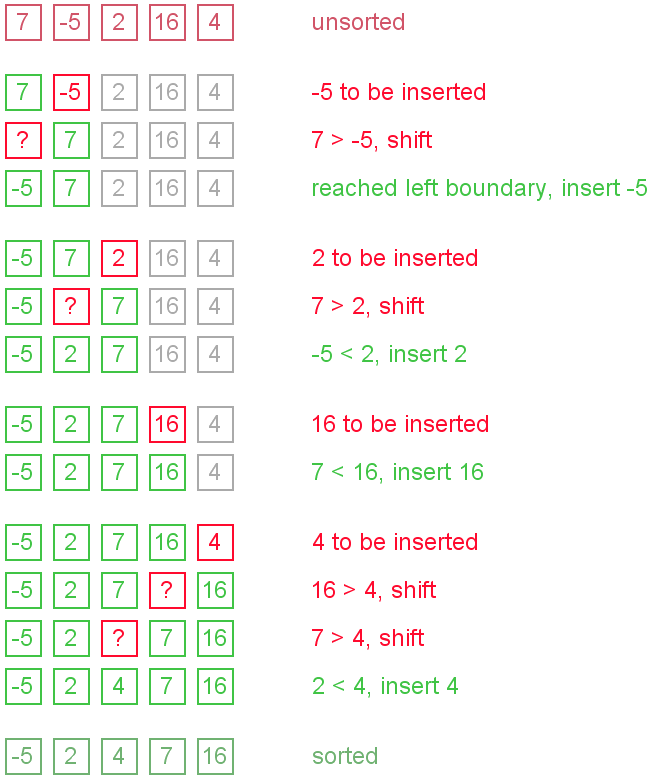## The ideas of insertion

The main operation of the algorithm is insertion. The task is to insert a value into the sorted part of the array. Let us see the variants of how we can do it.

"Sifting down" using swaps

The simplest way to insert next element into the sorted part is to sift it down, until it occupies correct position. Initially the element stays right after the sorted part. At each step algorithm compares the element with one before it and, if they stay in reversed order, swap them. Let us see an illustration.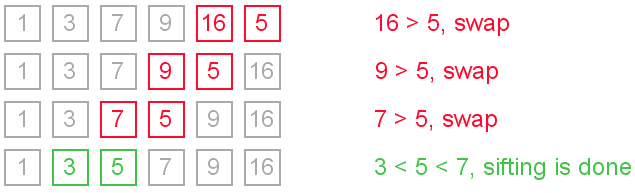This approach writes sifted element to temporary position many times. Next implementation eliminates those unnecessary writes.

We can modify previous algorithm, so it will write sifted element only to the final correct position. Let us see an illustration.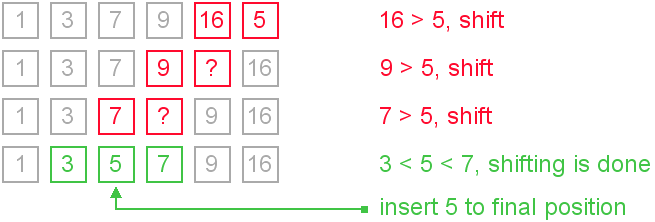It is the most commonly used modification of the insertion sort.

### Using binary search

It is reasonable to use binary search algorithm to find a proper place for insertion. This variant of the insertion sort is calledbinary insertion sort. After position for insertion is found, algorithm shifts the part of the array and inserts the element. This version has lower number of comparisons, but overall average complexity remains O(n2). From a practical point of view this improvement is not very important, because insertion sort is used on quite small data sets.

## Complexity analysis

Insertion sort's overall complexity is O(n2) on average, regardless of the method of insertion. On the almost sorted arrays insertion sort shows better performance, up to O(n) in case of applying insertion sort to a sorted array. Number of writes is O(n2) on average, but number of comparisons may vary depending on the insertion algorithm. It is O(n2) when shifting or swapping methods are used and O(n log n) for binary insertion sort.

From the point of view of practical application, an average complexity of the insertion sort is not so important. As it was mentioned above, insertion sort is applied to quite small data sets (from 8 to 12 elements). Therefore, first of all, a "practical performance" should be considered. In practice insertion sort outperforms most of the quadratic sorting algorithms, like selection sort or bubble sort.

## Insertion sort properties

• stable (insertion sort retains relative order of the same elements);
• in-place (requires constant amount of additional space);
• online (new elements can be added during the sort).

## Code snippets

We show the idea of insertion with shifts in Java implementation and the idea of insertion using python code snippet.

### Java implementation

void insertionSort(int[] arr) {

int i,j,newValue;

for(i=1;i<arr.length;i++){

newValue = arr[i];

j=i;

while(j>0&&arr[j-1]>newValue){

arr[j] = arr[j-1];

j--;

}

arr[j] = newValue;

}

### Python implementation

void insertionSort(L) {

for i in range(l,len(L)):

j = i

newValue = L[i]

while j > 0 and  L[j - 1] >L[j]:

L[j] = L[j - 1]

j = j-1

}

L[j] = newValue

}

}

posted @ 2011-05-08 12:24 ytl| 编辑 收藏

# Binary search algorithm

Generally, to find a value in unsorted array, we should look through elements of an array one by one, until searched value is found. In case of searched value is absent from array, we go through all elements. In average, complexity of such an algorithm is proportional to the length of the array.

Situation changes significantly, when array is sorted. If we know it, random access capability can be utilized very efficientlyto find searched value quick. Cost of searching algorithm reduces to binary logarithm of the array length. For reference, log2(1 000 000) ≈ 20. It means, that in worst case, algorithm makes 20 steps to find a value in sorted array of a million elements or to say, that it doesn't present it the array.

## Algorithm

Algorithm is quite simple. It can be done either recursively or iteratively:

1. get the middle element;
2. if the middle element equals to the searched value, the algorithm stops;
3. otherwise, two cases are possible:
• searched value is less, than the middle element. In this case, go to the step 1 for the part of the array, before middle element.
• searched value is greater, than the middle element. In this case, go to the step 1 for the part of the array, after middle element.
Now we should define, when iterations should stop. First case is when searched element is found. Second one is when subarray has no elements. In this case, we can conclude, that searched value doesn't present in the array.

## Examples

Example 1. Find 6 in {-1, 5, 6, 18, 19, 25, 46, 78, 102, 114}.

Step 1 (middle element is 19 > 6):     -1  5  6  18  19  25  46  78  102  114

Step 2 (middle element is 5 < 6):      -1  5  6  18  19  25  46  78  102  114

Step 3 (middle element is 6 == 6):     -1  5  6  18  19  25  46  78  102  114

Example 2. Find 103 in {-1, 5, 6, 18, 19, 25, 46, 78, 102, 114}.

Step 1 (middle element is 19 < 103):   -1  5  6  18  19  25  46  78  102  114

Step 2 (middle element is 78 < 103):   -1  5  6  18  19  25  46  78  102  114

Step 3 (middle element is 102 < 103):  -1  5  6  18  19  25  46  78  102  114

Step 4 (middle element is 114 > 103):  -1  5  6  18  19  25  46  78  102  114

Step 5 (searched value is absent):     -1  5  6  18  19  25  46  78  102  114

## Complexity analysis

Huge advantage of this algorithm is that it's complexity depends on the array size logarithmically in worst case. In practice it means, that algorithm will do at most log2(n) iterations, which is a very small number even for big arrays. It can be proved very easily. Indeed, on every step the size of the searched part is reduced by half. Algorithm stops, when there are no elements to search in. Therefore, solving following inequality in whole numbers:

n / 2iterations > 0

resulting in

iterations <= log2(n).

It means, that binary search algorithm time complexity is O(log2(n)).

## Code snippets.

You can see recursive solution for Java and iterative for python below.

### Java

int binarySearch(int[] array, int value, int left, int right) {

if (left > right)

return -1;

int middle = left + (right-left) / 2;

if (array[middle] == value)

return middle;

if (array[middle] > value)

return binarySearch(array, value, left, middle - 1);

else

return binarySearch(array, value, middle + 1, right);

}

### Python

def biSearch(L,e,first,last):

if last - first < 2: return L[first] == e or L[last] == e

mid = first + (last-first)/2

if L[mid] ==e: return True

if L[mid]> e :

return biSearch(L,e,first,mid-1)

return biSearch(L,e,mid+1,last)

posted @ 2011-05-06 18:11 ytl| 编辑 收藏

Merge sort is an O(n log ncomparison-based sorting algorithm. Most implementations produce a stable sort, meaning that the implementation preserves the input order of equal elements in the sorted output. It is a divide and conquer algorithm. Merge sort was invented by John von Neumann in 1945. A detailed description and analysis of bottom-up mergesort appeared in a report byGoldstine and Neumann as early as 1948
: 1, split the problem into several subproblem of the same type. 2,solove independetly. 3 combine those solutions

Python Implement

def mergeSort(L):

if len(L) < 2 :
return  L
middle = len(L)/2
left = mergeSort(L[:mddle])
right = mergeSort(L[middle:])
together = merge(left,right)

posted @ 2011-05-06 17:05 ytl| 编辑 收藏

# Algorithm to merge sorted arrays

In the article we present an algorithm for merging two sorted arrays. One can learn how to operate with several arrays and master read/write indices. Also, the algorithm has certain applications in practice, for instance in merge sort.

## Merge algorithm

Assume, that both arrays are sorted in ascending order and we want resulting array to maintain the same order. Algorithm to merge two arrays A[0..m-1] and B[0..n-1] into an array C[0..m+n-1] is as following:

1. Introduce read-indices ij to traverse arrays A and B, accordingly. Introduce write-index k to store position of the first free cell in the resulting array. By default i = j = k = 0.
2. At each step: if both indices are in range (i < m and j < n), choose minimum of (A[i], B[j]) and write it toC[k]. Otherwise go to step 4.
3. Increase k and index of the array, algorithm located minimal value at, by one. Repeat step 2.
4. Copy the rest values from the array, which index is still in range, to the resulting array.

## Enhancements

Algorithm could be enhanced in many ways. For instance, it is reasonable to check, if A[m - 1] < B orB[n - 1] < A. In any of those cases, there is no need to do more comparisons. Algorithm could just copy source arrays in the resulting one in the right order. More complicated enhancements may include searching for interleaving parts and run merge algorithm for them only. It could save up much time, when sizes of merged arrays differ in scores of times.

## Complexity analysis

Merge algorithm's time complexity is O(n + m). Additionally, it requires O(n + m) additional space to store resulting array.

## Code snippets

### Java implementation

// size of C array must be equal or greater than

// sum of A and B arrays' sizes

public void merge(int[] A, int[] B, int[] C) {

int i,j,k ;

i = 0;

j=0;

k=0;

m = A.length;

n = B.length;

while(i < m && j < n){

if(A[i]<= B[j]){

C[k] = A[i];

i++;

}else{

C[k] = B[j];

j++;

}

k++;

while(i<m){

C[k] = A[i]

i++;

k++;

}

while(j<n){

C[k] = B[j]

j++;

k++;

}

### Python  implementation

def merege(left,right):

result = []

i,j = 0

while i< len(left) and j < len(right):

if left[i]<= right[j]:

result.append(left[i])

i = i + 1

else:

result.append(right[j])

j = j + 1

while i< len(left):

result.append(left[i])

i = i + 1

while　j< len(right):

result.append(right[j])

j = j + 1

return result

MergSort:

import operator

def mergeSort(L, compare = operator.lt):
if len(L) < 2:
return L[:]
else:
middle = int(len(L)/2)
left = mergeSort(L[:middle], compare)
right= mergeSort(L[middle:], compare)
return merge(left, right, compare)

def merge(left, right, compare):
result = []
i, j = 0, 0

while i < len(left) and j < len(right):
if compare(left[i], right[j]):
result.append(left[i])
i += 1
else:
result.append(right[j])
j += 1
while i < len(left):
result.append(left[i])
i += 1
while j < len(right):
result.append(right[j])
j += 1
return result

posted @ 2011-05-06 16:55 ytl| 编辑 收藏

# Selection Sort

Selection sort is one of the O(n2) sorting algorithms, which makes it quite inefficient for sorting large data volumes. Selection sort is notable for its programming simplicity and it can over perform other sorts in certain situations (see complexity analysis for more details).

## Algorithm

The idea of algorithm is quite simple. Array is imaginary divided into two parts - sorted one and unsorted one. At the beginning, sorted part is empty, while unsorted one contains whole arrayAt every step, algorithm finds minimal element in the unsorted part and adds it to the end of the sorted one. When unsorted part becomes empty, algorithmstops.

When algorithm sorts an array, it swaps first element of unsorted part with minimal element and then it is included to the sorted part. This implementation of selection sort in not stable. In case of linked list is sorted, and, instead of swaps, minimal element is linked to the unsorted part, selection sort is stable.

Let us see an example of sorting an array to make the idea of selection sort clearer.

Example. Sort {5, 1, 12, -5, 16, 2, 12, 14} using selection sort.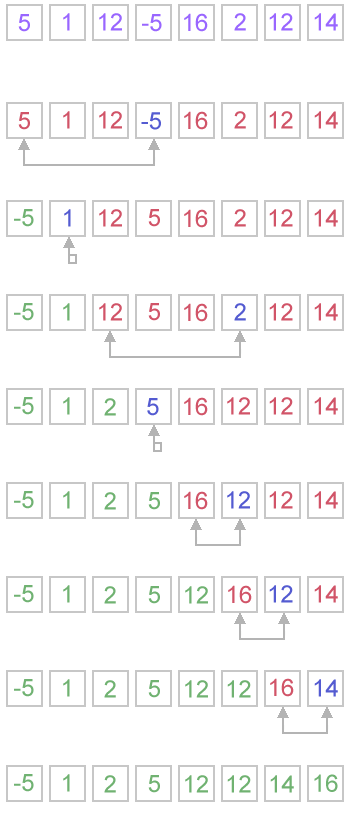## Complexity analysis

Selection sort stops, when unsorted part becomes empty. As we know, on every step number of unsorted elements decreased by one. Therefore, selection sort makes n steps (n is number of elements in array) of outer loop, before stop. Every step of outer loop requires finding minimum in unsorted part. Summing up, n + (n - 1) + (n - 2) + ... + 1, results in O(n2) number of comparisons. Number of swaps may vary from zero (in case of sorted array) to n - 1 (in case array was sorted in reversed order), which results in O(n) number of swaps. Overall algorithm complexity is O(n2).

Fact, that selection sort requires n - 1 number of swaps at most, makes it very efficient in situations, when write operation is significantly more expensive, than read operation.

## Code snippets

### Java

public void selectionSort(int[] arr) {

int i, j, minIndex, tmp;

int n = arr.length;

for (i = 0; i < n - 1; i++) {

minIndex = i;

for (j = i + 1; j < n; j++)

if (arr[j] < arr[minIndex])

minIndex = j;

if (minIndex != i) {

tmp = arr[i];

arr[i] = arr[minIndex];

arr[minIndex] = tmp;

}

}

}

### Python

for i in range(len(L)-1):
minIndex = i
minValue = L[i]
j = i + 1
while j< len(L):
if minValue > L[j]:
minIndex = j
minValue = L[j]
j += 1
if minIndex != i:
temp       = L[i]
L[i]       = L[minIndex]
L[minIndex] = temp

posted @ 2011-05-06 16:16 ytl| 编辑 收藏

# Bubble Sort

Bubble sort is a simple and well-known sorting algorithm. It is used in practice once in a blue moon and its main application is to make an introduction to the sorting algorithms. Bubble sort belongs to O(n2) sorting algorithms, which makes it quite inefficient for sorting large data volumes. Bubble sort is stable and adaptive.

## Algorithm

1. Compare each pair of adjacent elements from the beginning of an array and, if they are in reversed order, swap them.
2. If at least one swap has been done, repeat step 1.

You can imagine that on every step big bubbles float to the surface and stay there. At the step, when no bubble moves, sorting stops. Let us see an example of sorting an array to make the idea of bubble sort clearer.

Example. Sort {5, 1, 12, -5, 16} using bubble sort.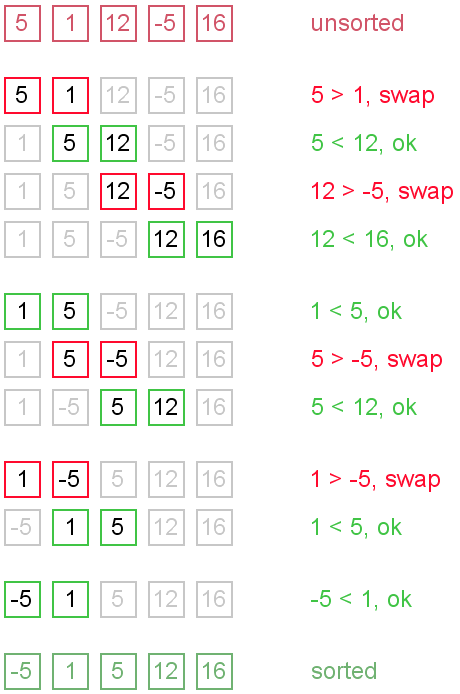## Complexity analysis

Average and worst case complexity of bubble sort is O(n2). Also, it makes O(n2) swaps in the worst case. Bubble sort is adaptive. It means that for almost sorted array it gives O(n) estimation. Avoid implementations, which don't check if the array is already sorted on every step (any swaps made). This check is necessary, in order to preserve adaptive property.

### Turtles and rabbits

One more problem of bubble sort is that its running time badly depends on the initial order of the elements. Big elements (rabbits) go up fast, while small ones (turtles) go down very slow. This problem is solved in the Cocktail sort.

Turtle example. Thought, array {2, 3, 4, 5, 1} is almost sorted, it takes O(n2) iterations to sort an array. Element {1} is a turtle.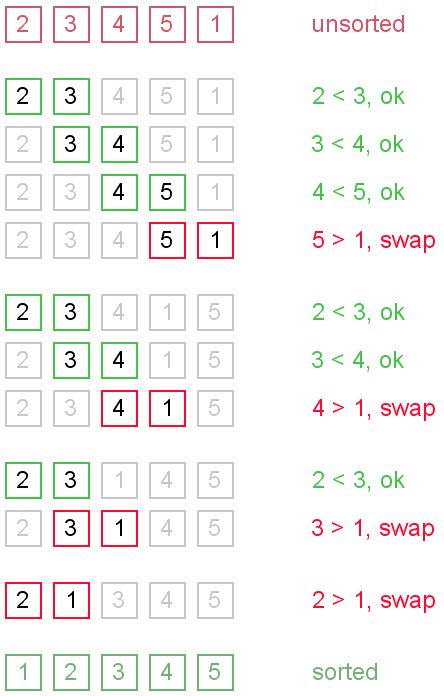Rabbit example. Array {6, 1, 2, 3, 4, 5} is almost sorted too, but it takes O(n) iterations to sort it. Element {6} is a rabbit. This example demonstrates adaptive property of the bubble sort.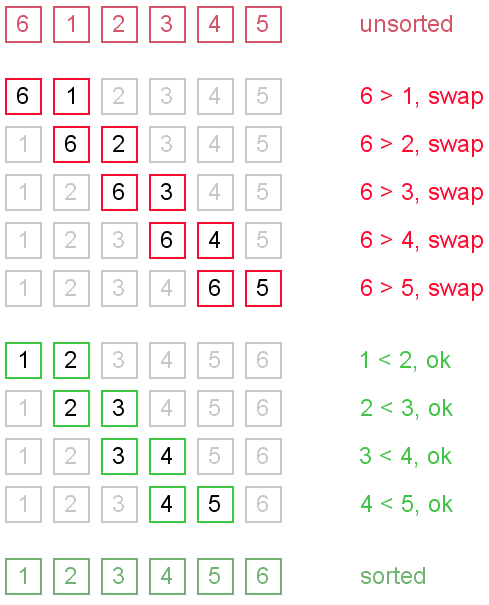## Code snippets

There are several ways to implement the bubble sort. Notice, that "swaps" check is absolutely necessary, in order to preserve adaptive property.

### Java

public void bubbleSort(int[] arr) {

boolean swapped = true;

int j = 0;

int tmp;

while (swapped) {

swapped = false;

j++;

for (int i = 0; i < arr.length - j; i++) {

if (arr[i] > arr[i + 1]) {

tmp = arr[i];

arr[i] = arr[i + 1];

arr[i + 1] = tmp;

swapped = true;

}

}

}

}

### Python

def bubbleSort(L) :

swapped = True;

while swapped:

swapped = False

for i in range(len(L)-1):

if L[i]>L[i+1]:

temp = L[i]

L[i] = L[i+1]

L[i+1] = temp

swapped = True

posted @ 2011-05-06 16:03 ytl| 编辑 收藏

JNDI : Java Naming and Directory Interface (JNDI)
JNDI works in concert with other technologies in the Java Platform, Enterprise Edition (Java EE) to organize and locate components in a distributed computing environment.

Tomcat 6.0 的数据源配置

1，在Tomcat中配置：
tomcat 安装目录下的conf的context.xml 的
<Context></Context>中

<Resource  name="jdbc/tango"
auth
="Container"
type
="javax.sql.DataSource"
maxActive
="20"
maxIdel
="10"
maxWait
="1000"
="root"
="root"
driverClassName
="com.mysql.jdbc.Driver"        url="jdbc:mysql://localhost:3306/tango"

>

</Resource>

name 表示指定的jndi名称
auth 表示认证方式，一般为Container
type 表示数据源床型，使用标准的javax.sql.DataSource
maxActive 表示连接池当中最大的数据库连接
maxIdle 表示最大的空闲连接数
maxWait 当池的数据库连接已经被占用的时候，最大等待时间
driverClassName 表示JDBC DRIVER
url 表示数据库URL地址

factory="org.apache.commons.dbcp.BasicDataSourceFactory"

2在项目的中配置：

2.1  使用自己的DBCP数据源

factory="org.apache.commons.dbcp.BasicDataSourceFactory"

factory="org.apache.tomcat.dbcp.dbcp.BasicDataSourceFactory" 改为
factory="org.apache.commons.dbcp.BasicDataSourceFactory"

2.2 使用Tomcat 自带的DBCP数据源

factory="org.apache.tomcat.dbcp.dbcp.BasicDataSourceFactory"

JNDI使用示例代码：Context initContext;try
{Context context
=new
InitialContext();DataSource ds
=(DataSource) context.lookup("java:/comp/env/jdbc/tango"
);//
"java:/comp/env/"是固定写法，后面接的是 context.xml中的Resource中name属性的值Connection conn = ds.getConnection();Statement stmt
=
conn.createStatement();ResultSet set
= stmt.executeQuery("SELECT id,name,age FROM user_lzy"
);while(set.next())
{System.out.println(set.getString(
"name"
));}//etc.}
catch (NamingException e) {// TODO Auto-generated catch blocke.printStackTrace();}
catch (SQLException e) {// TODO Auto-generated catch blocke.printStackTrace();}

posted @ 2009-04-14 17:10 ytl 阅读(8031) | 评论 (6)编辑 收藏

摘要: 欧几里德算法  阅读全文

posted @ 2013-03-21 09:39 ytl 阅读(281) | 评论 (0)编辑 收藏

不同的平台，内存模型是不一样的，但是jvm的内存模型规范是统一的。其实java的多线程并发问题最终都会反映在java的内存模型上，所谓线程安全无 非是要控制多个线程对某个资源的有序访问或修改。总结java的内存模型，要解决两个主要的问题：可见性和有序性。我们都知道计算机有高速缓存的存在，处 理器并不是每次处理数据都是取内存的。JVM定义了自己的内存模型，屏蔽了底层平台内存管理细节，对于java开发人员，要清楚在jvm内存模型的基础 上，如果解决多线程的可见性和有序性。
那么，何谓可见性？ 多个线程之间是不能互相传递数据通信的，它们之间的沟通只能通过共享变量来进行。Java内存模型（JMM）规定了jvm有主内存，主内存是多个线程共享 的。当new一个对象的时候，也是被分配在主内存中，每个线程都有自己的工作内存，工作内存存储了主存的某些对象的副本，当然线程的工作内存大小是有限制 的。当线程操作某个对象时，执行顺序如下：
(2) 执行代码，改变共享变量值 (use and assign)
(3) 用工作内存数据刷新主存相关内容 (store and write)

Java代码
for(int i=0;i<10;i++)
a++;

1 从主存中读取变量x副本到工作内存
2 给x加1
3 将x加1后的值写回主 存

1 从主存中读取变量x副本到工作内存
2 给x减1
3 将x减1后的值写回主存

1：线程a从主存读取x副本到工作内存，工作内存中x值为10
2：线程b从主存读取x副本到工作内存，工作内存中x值为10
3：线程a将工作内存中x加1，工作内存中x值为11
4：线程a将x提交主存中，主存中x为11
5：线程b将工作内存中x值减1，工作内存中x值为9
6：线程b将x提交到中主存中，主存中x为

jvm的内存模型之eden区

可能 很多人都觉得莫名其妙，说JVM的内存模型，怎么会扯到cpu上去呢？在此，我认为很有必要阐述下，免 得很多人看得不明不白的。先抛开java虚拟机不谈，我们都知道，现在的计算机，cpu在计算的时候，并不总是从内存读取数据，它的数据读取顺序优先级 是：寄存器－高速缓存－内存。线程耗费的是CPU，线程计算的时候，原始的数据来自内存，在计算过程中，有些数据可能被频繁读取，这些数据被存储在寄存器 和高速缓存中，当线程计算完后，这些缓存的数据在适当的时候应该写回内存。当个多个线程同时读写某个内存数据时，就会产生多线程并发问题，涉及到三个特 性：原子性，有序性，可见性。在《线程安全总结》这篇文章中，为了理解方便，我把原子性和有序性统一叫做“多线程执行有序性”。支持多线程的平台都会面临 这种问题，运行在多线程平台上支持多线程的语言应该提供解决该问题的方案。

synchronized, volatile,锁机制（如同步块，就绪队 列，阻塞队列）等等。这些方案只是语法层面的，但我们要从本质上去理解它，不能仅仅知道一个 synchronized 可以保证同步就完了。   在这里我说的是jvm的内存模型，是动态的，面向多线程并发的，沿袭JSL的“working memory”的说法，只是不想牵扯到太多底层细节，因为 《线程安全总结》这篇文章意在说明怎样从语法层面去理解java的线程同步，知道各个关键字的使用场 景。

1.程序计数器

2.线程栈

3.本地方法栈
4.堆

5.方法区

6.常量池
Class文件中除了有类的版本、字段、方法、接口等描述等信息外，还有一项信息是常量表(constant_pool table)，用于存放编译期已可知的常量，这部分内容将在类加载后进入方法区（永久代）存放。但是Java语言并不要求常量一定只有编译期预置入Class的常量表的内容才能进入方法区常量池，运行期间也可将新内容放入常量池（最典型的String.intern()方法）。

posted @ 2012-03-01 18:12 ytl 阅读(956) | 评论 (0)编辑 收藏

ArrayList
关于Java中的transient，volatile和strictfp关键字 http://www.iteye.com/topic/52957
(1), ArrayList底层使用Object数据实现， private transient Object[] elementData;且在使用不带参数的方式实例化时，生成数组默认的长度是10。
//ensureCapacityInternal判断添加新元素是否需要重新扩大数组的长度，需要则扩否则不
ensureCapacityInternal(size + 1);  // 此为JDK7调用的方法 JDK5里面使用的ensureCapacity方法
elementData[size++] = e; //把对象插入数组，同时把数组存储的数据长度size加1
return true;
}
JDK 7中 ensureCapacityInternal实现
private void ensureCapacityInternal(int minCapacity) {
modCount++;修改次数
// overflow-conscious code
if (minCapacity - elementData.length > 0)
grow(minCapacity);//如果需要扩大数组长度
}
/**
* The maximum size of array to allocate. --申请新数组最大长度
* Some VMs reserve some header words in an array.
* Attempts to allocate larger arrays may result in
* OutOfMemoryError: Requested array size exceeds VM limit  --如果申请的数组占用的内心大于JVM的限制抛出异常
*/
private static final int MAX_ARRAY_SIZE = Integer.MAX_VALUE - 8;//为什么减去8看注释第2行
/**
* Increases the capacity to ensure that it can hold at least the
* number of elements specified by the minimum capacity argument.
*
* @param minCapacity the desired minimum capacity
*/
private void grow(int minCapacity) {
// overflow-conscious code
int oldCapacity = elementData.length;
int newCapacity = oldCapacity + (oldCapacity >> 1); //新申请的长度为old的3/2倍同时使用位移运算更高效，JDK5中： (oldCapacity *3)/2+1
if (newCapacity - minCapacity < 0)
newCapacity = minCapacity;
if (newCapacity - MAX_ARRAY_SIZE > 0) //你懂的
newCapacity = hugeCapacity(minCapacity);
// minCapacity is usually close to size, so this is a win:
elementData = Arrays.copyOf(elementData, newCapacity);
}
//可以申请的最大长度
private static int hugeCapacity(int minCapacity) {
if (minCapacity < 0) // overflow
throw new OutOfMemoryError();
return (minCapacity > MAX_ARRAY_SIZE) ?
Integer.MAX_VALUE :
MAX_ARRAY_SIZE;
}

posted @ 2011-09-24 15:30 ytl| 编辑 收藏

摘要:   阅读全文

posted @ 2011-08-07 11:02 ytl| 编辑 收藏

# Quicksort

Quicksort is a fast sorting algorithm, which is used not only for educational purposes, but widely applied in practice. On the average, it has O(n log n) complexity, making quicksort suitable for sorting big data volumes. The idea of the algorithm is quite simple and once you realize it, you can write quicksort as fast as bubble sort.

## Algorithm

The divide-and-conquer strategy is used in quicksort. Below the recursion step is described:
1. Choose a pivot value. We take the value of the middle element as pivot value, but it can be any value, which is in range of sorted values, even if it doesn't present in the array.
2. Partition. Rearrange elements in such a way, that all elements which are lesser than the pivot go to the left part of the array and all elements greater than the pivot, go to the right part of the array. Values equal to the pivot can stay in any part of the array. Notice, that array may be divided in non-equal parts.
3. Sort both parts. Apply quicksort algorithm recursively to the left and the right parts.

### Partition algorithm in detail

There are two indices i and j and at the very beginning of the partition algorithm i points to the first element in the array andj points to the last one. Then algorithm moves i forward, until an element with value greater or equal to the pivot is found. Index j is moved backward, until an element with value lesser or equal to the pivot is found. If i ≤ j then they are swapped and i steps to the next position (i + 1), j steps to the previous one (j - 1). Algorithm stops, when i becomes greater than j.

After partition, all values before i-th element are less or equal than the pivot and all values after j-th element are greater or equal to the pivot.

Example. Sort {1, 12, 5, 26, 7, 14, 3, 7, 2} using quicksort.Notice, that we show here only the first recursion step, in order not to make example too long. But, in fact, {1, 2, 5, 7, 3} and {14, 7, 26, 12} are sorted then recursively.

## Why does it work?

On the partition step algorithm divides the array into two parts and every element a from the left part is less or equal than every element b from the right part. Also a and b satisfy a ≤ pivot ≤ b inequality. After completion of the recursion calls both of the parts become sorted and, taking into account arguments stated above, the whole array is sorted.

## Complexity analysis

On the average quicksort has O(n log n) complexity, but strong proof of this fact is not trivial and not presented here. Still, you can find the proof in . In worst case, quicksort runs O(n2) time, but on the most "practical" data it works just fine and outperforms other O(n log n) sorting algorithms.

## Code snippets

### Java

int partition(int arr[], int left, int right)

{

int i = left;

int j = right;

int temp;

int  pivot = arr[(left+right)>>1];

while(i<=j){

while(arr[i]>=pivot){

i++;

}

while(arr[j]<=pivot){

j--;

}

if(i<=j){

temp = arr[i];

arr[i] = arr[j];

arr[j] = temp;

i++;

j--;

}

}

return i

}

void quickSort(int arr[], int left, int right) {

int index = partition(arr, left, right);

if(left<index-1){

quickSort(arr,left,index-1);

}

if(index<right){

quickSort(arr,index,right);

}

}

### python

def quickSort(L,left,right) {

i = left

j = right

if right-left <=1:

return L

pivot = L[(left + right) >>1];

/* partition */

while (i <= j) {

while (L[i] < pivot)

i++;

while (L[j] > pivot)

j--;

if (i <= j) {

L[i],L[j] = L[j],L[i]

i++;

j--;

}

};

/* recursion */

if (left < j)

quickSort(Lleftj);

if (i < right)

quickSort(Liright);

}

posted @ 2011-05-08 14:13 ytl| 编辑 收藏

# Insertion Sort

Insertion sort belongs to the O(n2) sorting algorithms. Unlike many sorting algorithms with quadratic complexity, it is actually applied in practice for sorting small arrays of data. For instance, it is used to improve quicksort routine. Some sources notice, that people use same algorithm ordering items, for example, hand of cards.

## Algorithm

Insertion sort algorithm somewhat resembles selection sort. Array is imaginary divided into two parts - sorted one andunsorted one. At the beginning, sorted part contains first element of the array and unsorted one contains the rest. At every step, algorithm takes first element in the unsorted part and inserts it to the right place of the sorted one. Whenunsorted part becomes empty, algorithm stops. Sketchy, insertion sort algorithm step looks like this:becomesThe idea of the sketch was originaly posted here.

Let us see an example of insertion sort routine to make the idea of algorithm clearer.

Example. Sort {7, -5, 2, 16, 4} using insertion sort.## The ideas of insertion

The main operation of the algorithm is insertion. The task is to insert a value into the sorted part of the array. Let us see the variants of how we can do it.

"Sifting down" using swaps

The simplest way to insert next element into the sorted part is to sift it down, until it occupies correct position. Initially the element stays right after the sorted part. At each step algorithm compares the element with one before it and, if they stay in reversed order, swap them. Let us see an illustration.This approach writes sifted element to temporary position many times. Next implementation eliminates those unnecessary writes.

We can modify previous algorithm, so it will write sifted element only to the final correct position. Let us see an illustration.It is the most commonly used modification of the insertion sort.

### Using binary search

It is reasonable to use binary search algorithm to find a proper place for insertion. This variant of the insertion sort is calledbinary insertion sort. After position for insertion is found, algorithm shifts the part of the array and inserts the element. This version has lower number of comparisons, but overall average complexity remains O(n2). From a practical point of view this improvement is not very important, because insertion sort is used on quite small data sets.

## Complexity analysis

Insertion sort's overall complexity is O(n2) on average, regardless of the method of insertion. On the almost sorted arrays insertion sort shows better performance, up to O(n) in case of applying insertion sort to a sorted array. Number of writes is O(n2) on average, but number of comparisons may vary depending on the insertion algorithm. It is O(n2) when shifting or swapping methods are used and O(n log n) for binary insertion sort.

From the point of view of practical application, an average complexity of the insertion sort is not so important. As it was mentioned above, insertion sort is applied to quite small data sets (from 8 to 12 elements). Therefore, first of all, a "practical performance" should be considered. In practice insertion sort outperforms most of the quadratic sorting algorithms, like selection sort or bubble sort.

## Insertion sort properties

• stable (insertion sort retains relative order of the same elements);
• in-place (requires constant amount of additional space);
• online (new elements can be added during the sort).

## Code snippets

We show the idea of insertion with shifts in Java implementation and the idea of insertion using python code snippet.

### Java implementation

void insertionSort(int[] arr) {

int i,j,newValue;

for(i=1;i<arr.length;i++){

newValue = arr[i];

j=i;

while(j>0&&arr[j-1]>newValue){

arr[j] = arr[j-1];

j--;

}

arr[j] = newValue;

}

### Python implementation

void insertionSort(L) {

for i in range(l,len(L)):

j = i

newValue = L[i]

while j > 0 and  L[j - 1] >L[j]:

L[j] = L[j - 1]

j = j-1

}

L[j] = newValue

}

}

posted @ 2011-05-08 12:24 ytl| 编辑 收藏

# Binary search algorithm

Generally, to find a value in unsorted array, we should look through elements of an array one by one, until searched value is found. In case of searched value is absent from array, we go through all elements. In average, complexity of such an algorithm is proportional to the length of the array.

Situation changes significantly, when array is sorted. If we know it, random access capability can be utilized very efficientlyto find searched value quick. Cost of searching algorithm reduces to binary logarithm of the array length. For reference, log2(1 000 000) ≈ 20. It means, that in worst case, algorithm makes 20 steps to find a value in sorted array of a million elements or to say, that it doesn't present it the array.

## Algorithm

Algorithm is quite simple. It can be done either recursively or iteratively:

1. get the middle element;
2. if the middle element equals to the searched value, the algorithm stops;
3. otherwise, two cases are possible:
• searched value is less, than the middle element. In this case, go to the step 1 for the part of the array, before middle element.
• searched value is greater, than the middle element. In this case, go to the step 1 for the part of the array, after middle element.
Now we should define, when iterations should stop. First case is when searched element is found. Second one is when subarray has no elements. In this case, we can conclude, that searched value doesn't present in the array.

## Examples

Example 1. Find 6 in {-1, 5, 6, 18, 19, 25, 46, 78, 102, 114}.

Step 1 (middle element is 19 > 6):     -1  5  6  18  19  25  46  78  102  114

Step 2 (middle element is 5 < 6):      -1  5  6  18  19  25  46  78  102  114

Step 3 (middle element is 6 == 6):     -1  5  6  18  19  25  46  78  102  114

Example 2. Find 103 in {-1, 5, 6, 18, 19, 25, 46, 78, 102, 114}.

Step 1 (middle element is 19 < 103):   -1  5  6  18  19  25  46  78  102  114

Step 2 (middle element is 78 < 103):   -1  5  6  18  19  25  46  78  102  114

Step 3 (middle element is 102 < 103):  -1  5  6  18  19  25  46  78  102  114

Step 4 (middle element is 114 > 103):  -1  5  6  18  19  25  46  78  102  114

Step 5 (searched value is absent):     -1  5  6  18  19  25  46  78  102  114

## Complexity analysis

Huge advantage of this algorithm is that it's complexity depends on the array size logarithmically in worst case. In practice it means, that algorithm will do at most log2(n) iterations, which is a very small number even for big arrays. It can be proved very easily. Indeed, on every step the size of the searched part is reduced by half. Algorithm stops, when there are no elements to search in. Therefore, solving following inequality in whole numbers:

n / 2iterations > 0

resulting in

iterations <= log2(n).

It means, that binary search algorithm time complexity is O(log2(n)).

## Code snippets.

You can see recursive solution for Java and iterative for python below.

### Java

int binarySearch(int[] array, int value, int left, int right) {

if (left > right)

return -1;

int middle = left + (right-left) / 2;

if (array[middle] == value)

return middle;

if (array[middle] > value)

return binarySearch(array, value, left, middle - 1);

else

return binarySearch(array, value, middle + 1, right);

}

### Python

def biSearch(L,e,first,last):

if last - first < 2: return L[first] == e or L[last] == e

mid = first + (last-first)/2

if L[mid] ==e: return True

if L[mid]> e :

return biSearch(L,e,first,mid-1)

return biSearch(L,e,mid+1,last)

posted @ 2011-05-06 18:11 ytl| 编辑 收藏

Merge sort is an O(n log ncomparison-based sorting algorithm. Most implementations produce a stable sort, meaning that the implementation preserves the input order of equal elements in the sorted output. It is a divide and conquer algorithm. Merge sort was invented by John von Neumann in 1945. A detailed description and analysis of bottom-up mergesort appeared in a report byGoldstine and Neumann as early as 1948
: 1, split the problem into several subproblem of the same type. 2,solove independetly. 3 combine those solutions

Python Implement

def mergeSort(L):

if len(L) < 2 :
return  L
middle = len(L)/2
left = mergeSort(L[:mddle])
right = mergeSort(L[middle:])
together = merge(left,right)

posted @ 2011-05-06 17:05 ytl| 编辑 收藏

# Algorithm to merge sorted arrays

In the article we present an algorithm for merging two sorted arrays. One can learn how to operate with several arrays and master read/write indices. Also, the algorithm has certain applications in practice, for instance in merge sort.

## Merge algorithm

Assume, that both arrays are sorted in ascending order and we want resulting array to maintain the same order. Algorithm to merge two arrays A[0..m-1] and B[0..n-1] into an array C[0..m+n-1] is as following:

1. Introduce read-indices ij to traverse arrays A and B, accordingly. Introduce write-index k to store position of the first free cell in the resulting array. By default i = j = k = 0.
2. At each step: if both indices are in range (i < m and j < n), choose minimum of (A[i], B[j]) and write it toC[k]. Otherwise go to step 4.
3. Increase k and index of the array, algorithm located minimal value at, by one. Repeat step 2.
4. Copy the rest values from the array, which index is still in range, to the resulting array.

## Enhancements

Algorithm could be enhanced in many ways. For instance, it is reasonable to check, if A[m - 1] < B orB[n - 1] < A. In any of those cases, there is no need to do more comparisons. Algorithm could just copy source arrays in the resulting one in the right order. More complicated enhancements may include searching for interleaving parts and run merge algorithm for them only. It could save up much time, when sizes of merged arrays differ in scores of times.

## Complexity analysis

Merge algorithm's time complexity is O(n + m). Additionally, it requires O(n + m) additional space to store resulting array.

## Code snippets

### Java implementation

// size of C array must be equal or greater than

// sum of A and B arrays' sizes

public void merge(int[] A, int[] B, int[] C) {

int i,j,k ;

i = 0;

j=0;

k=0;

m = A.length;

n = B.length;

while(i < m && j < n){

if(A[i]<= B[j]){

C[k] = A[i];

i++;

}else{

C[k] = B[j];

j++;

}

k++;

while(i<m){

C[k] = A[i]

i++;

k++;

}

while(j<n){

C[k] = B[j]

j++;

k++;

}

### Python  implementation

def merege(left,right):

result = []

i,j = 0

while i< len(left) and j < len(right):

if left[i]<= right[j]:

result.append(left[i])

i = i + 1

else:

result.append(right[j])

j = j + 1

while i< len(left):

result.append(left[i])

i = i + 1

while　j< len(right):

result.append(right[j])

j = j + 1

return result

MergSort:

import operator

def mergeSort(L, compare = operator.lt):
if len(L) < 2:
return L[:]
else:
middle = int(len(L)/2)
left = mergeSort(L[:middle], compare)
right= mergeSort(L[middle:], compare)
return merge(left, right, compare)

def merge(left, right, compare):
result = []
i, j = 0, 0

while i < len(left) and j < len(right):
if compare(left[i], right[j]):
result.append(left[i])
i += 1
else:
result.append(right[j])
j += 1
while i < len(left):
result.append(left[i])
i += 1
while j < len(right):
result.append(right[j])
j += 1
return result

posted @ 2011-05-06 16:55 ytl| 编辑 收藏

# Selection Sort

Selection sort is one of the O(n2) sorting algorithms, which makes it quite inefficient for sorting large data volumes. Selection sort is notable for its programming simplicity and it can over perform other sorts in certain situations (see complexity analysis for more details).

## Algorithm

The idea of algorithm is quite simple. Array is imaginary divided into two parts - sorted one and unsorted one. At the beginning, sorted part is empty, while unsorted one contains whole arrayAt every step, algorithm finds minimal element in the unsorted part and adds it to the end of the sorted one. When unsorted part becomes empty, algorithmstops.

When algorithm sorts an array, it swaps first element of unsorted part with minimal element and then it is included to the sorted part. This implementation of selection sort in not stable. In case of linked list is sorted, and, instead of swaps, minimal element is linked to the unsorted part, selection sort is stable.

Let us see an example of sorting an array to make the idea of selection sort clearer.

Example. Sort {5, 1, 12, -5, 16, 2, 12, 14} using selection sort.## Complexity analysis

Selection sort stops, when unsorted part becomes empty. As we know, on every step number of unsorted elements decreased by one. Therefore, selection sort makes n steps (n is number of elements in array) of outer loop, before stop. Every step of outer loop requires finding minimum in unsorted part. Summing up, n + (n - 1) + (n - 2) + ... + 1, results in O(n2) number of comparisons. Number of swaps may vary from zero (in case of sorted array) to n - 1 (in case array was sorted in reversed order), which results in O(n) number of swaps. Overall algorithm complexity is O(n2).

Fact, that selection sort requires n - 1 number of swaps at most, makes it very efficient in situations, when write operation is significantly more expensive, than read operation.

## Code snippets

### Java

public void selectionSort(int[] arr) {

int i, j, minIndex, tmp;

int n = arr.length;

for (i = 0; i < n - 1; i++) {

minIndex = i;

for (j = i + 1; j < n; j++)

if (arr[j] < arr[minIndex])

minIndex = j;

if (minIndex != i) {

tmp = arr[i];

arr[i] = arr[minIndex];

arr[minIndex] = tmp;

}

}

}

### Python

for i in range(len(L)-1):
minIndex = i
minValue = L[i]
j = i + 1
while j< len(L):
if minValue > L[j]:
minIndex = j
minValue = L[j]
j += 1
if minIndex != i:
temp       = L[i]
L[i]       = L[minIndex]
L[minIndex] = temp

posted @ 2011-05-06 16:16 ytl| 编辑 收藏